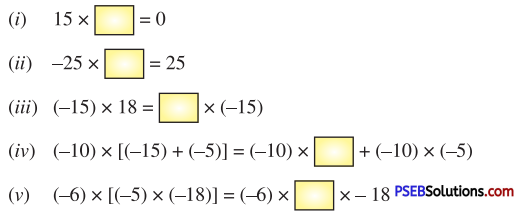# PSEB 7th Class Maths Solutions Chapter 1 Integers Ex 1.3

Punjab State Board PSEB 7th Class Maths Book Solutions Chapter 1 Integers Ex 1.3 Textbook Exercise Questions and Answers.

## PSEB Solutions for Class 7 Maths Chapter 1 Integers Ex 1.3

1. Find the product of :
(i) (-15) × 0
(ii) (-35) × 1
(iii) (-13) × (-12)
(iv) (-20) × 16
(v) (-15) × (-4) × (-5)
(vi) (-8) × (-5) × 9
(vii) (-2) × (-5) × (-4) × (-10)
(viii) (-8) × 0 + [(-5) × (-4)]
(i) (-15) × 0 = 0.
(ii) (-35) × 1 = -35.
(iii) (-13) × (-12) = 156.
(iv) (-20) × 16 = -(20 × 16) = -320

(v) (-15) × (-4) × (-5) = (-15 × -4) × (-5)
= 60 × (-5)
= -(60 × 5)
= -300.

(vi) (-8) × (-5) × 9
= [(-8) × (-5)] × 9
= 40 × 9 = 360.

(vii) (-2) × (-5) × (-4) × (-10)
= [(-2) × (-5)] × [(-4) × (-10)]
= 10 × 40 = 400.

(viii) (-8) × 0 + [(-5) × (-4)]
= [(-8) × 0] + [(-5) × (-4)]
= 0 + 20 = 20.2.
Question (i).
Verify : 15 × [9 + (-6)] = (15 × 9) + (15 × (-6)]
L.H.S. = 15 × [9 + (-6)]
= 15 × [9 – 6]
= 15 × 3 = 45.
R.H.S. = (15 × 9) + [(15 × (-6)]
= 135 + (-90)
= 135 – 90 = 45
∴ L.H.S = R.H.S.

Question (ii).
Verify : 18 × [(-5) + (-4)] = [18 × (-5)] + [18 × (-4)]
L.H.S. = 18 × [(-5) + (-4)]
= 18 × (-9)
= -162.
R.H.S. = [(18) × (-5)] + [18 × (-4)] = (-90) + (-72)
= – 162.
∴ L.H.S. = R.H.S.

3. Fill in the blanks :(i) 15 × 0 = 0.
(ii) -25 × -1 = 25.
(iii) (-15) × 18 = 18 × (-15)
(iv) (-10) × [(-15) + (-5)] = (-10) × -15 + (-10) × (-5)
(v) (-6) × [(-5) × (-18)] = (-6) × -5 × -18.4. Find product using properties :

Question (i).
15 × (-20) + (-20) × (-5)
15 × (-20) + (-20) × (-5)
= (-20) × [15 + (-5)]
= (-20) × [15 – 5]
= (-20) × (10)
= -200.

Question (ii).
(15 × 8) × 50
(15 × 8) × 50
= 120 × 50
= 6000.

Question (iii).
8 × (40 – 5)
8 × (40 – 5)
= 8 × 40 – 8 × 5
= 320 – 40
= 280.

Question (iv).
510 × (-45) + (-510) × 55
510 × (-45) + (-510) × 55
= 510 [(-45) + (-55)]
= 510 [-100]
= -51000.

5. In a class test containing 15 questions, 2 marks are awarded for every correct answer and (-1) mark awarded for every incorrect answer and 0 mark for questions not attempted.
(i) Kritika gets 5 correct and 10 incorrect answers. What is her score ?
(ii) Rohan gets 7 correct and 7 incorrect answers out of 14 questions he attempted. What is his score ?
(i) Marks given for one correct answer = 2
Marks given for 5 correct answers = 2 × 5 = 10
Marks given for one incorrect answer = -1
Marks given for 10 incorrect answers = -1 × 10 = -10
Total marks Kritika scored in the test = 10 + (-10)
= 10 – 10 = 0.

(ii) Marks given for one correct answer = 2
Marks given for 7 correct answers = 2 × 7 = 14
Marks given for one incorrect answer = -1
Marks given for 7 incorrect answers = – 1 × 7 = -7
Total marks Rohan scored in the test = 14 + (-7)
= 14 – 7 = 7.6. Multiple Choice Questions :

Question (i).
(-19) – (13) is equal to
(a) -32
(b) 6
(c) -6
(d) None of these.
(c) -6

Question (ii).
(-6) × (-5) × 0 is equal to :
(a) 0
(b) -6
(c) -5
(d) 30.
(a) 0

(iii) 0 ÷ (-10) is equal to :
(a) 0
(b) -1
(c) -10
(d) None of these.
(a) 0

(iv) (-33) × 102 + (-33) × (-2) is equal to :
(a) 3300
(b) -3300
(c) 3432
(d) -3432.